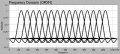# Radio frequency - subcarrier and its relation bandwidth!

#### JimmyCho

Joined Aug 1, 2020
109
Hi guys,
I understand what does the subcarrier mean but Im trying to understand what does it mean that subcarrier has for instance
10MHZ bandwidth? does it mean it's capable to carry / load data that the received signal (the signal of subcarrier+ data ) with 10MHZ?

assume I've data to transmit over that subcarrier (its bandwidth is 10MHZ) ..so the received signal has bandwidth 10MHZ?

I'm trying to understand the relation of saying subcarrier and has bandwidth 10MHz ..what does it mean actually? ..ofcourse if we have more than subcarrier..assume 4 subcarriers then bandwidth will be higher ..but what does that mean? I'm trying to understand the concept of that subcarrier has bandwidth and how it helps me / benefit me? maybe if bandwidth is more then I can load more data on that subcarrier for transmitting?

Im asking this in aspect of mobile communication ..assume I have transmitter and receiver.
assume I have subcarrier with bandwidth 10MHZ and I want to load on it data (data that I want to transmit) then I transmit that signal (subcarrier + data) to the receiver ..
here for instance I don't know what's the meaning or the benefit of having bandwidth 10MHZ or 9MHZ or whatever .. I'm trying to understand the meaning of bandwidth on the subcarrier.

Appreciated for any help, thanks alot!

Last edited:

#### Delta Prime

Joined Nov 15, 2019
1,311
Hello there againin one week's time you will become an expert with the types of questions you are asking.
In time domain :
1) 10 ms long with 10 subframes each of 1 ms.
2) Each subframe has two slots of 0.5 ms each & each slot has 7 orthogonal symbols.
In freq domain :
1) 72 subcarriers each of 15khz bandwidth & grouped into 6 RBs (PRBs).
Collectively :
1) An entity consisting of a subframe (1ms) of time domain & 12 subcarriers is known as Scheduling Block (SB).
2) An entity consisting of a slot (0.5ms) of time domain & 12 subcarriers is known as Resource Block (RB).
3) An entity consisting of a orthogonal symbol (0.071428 ms) & one subcarrier (15khz) is known as Resource Element (RE).
So a LTE radio frame consists of 10 subframes (i.e. total 1 ms) in time domain & 72 subcarriers (i.e. total 1080 Khz) in freq domain#### DickCappels

Joined Aug 21, 2008
10,067
What kind of modulation are you thinking of?

#### crutschow

Joined Mar 14, 2008
32,901
assume I've data to transmit over that subcarrier (its bandwidth is 10MHZ) ..so the received signal has bandwidth 10MHZ?
You seem to be confusing the subcarrier frequency with the subcarrier bandwidth.
They are not the same.
The subcarrier is a single frequency with no bandwidth.
The bandwidth is determined by the modulation frequency and the type of modulation.
Thus, for example, with simple AM modulation by a 1kHz sinewave, the bandwidth of the subcarrier would be 2kHz around the subcarrier frequency.

Last edited:

#### JimmyCho

Joined Aug 1, 2020
109
You seem to be confusing the subcarrier frequency with the subcarrier bandwidth.
They are not the same.
The subcarrier is a single frequency with no bandwidth.
The bandwidth is determined by the modulation frequency and the type of modulation.
Thus, for example, with simple AM modulation by a 1kHz sinewave, the bandwidth of the subcarrier would be 2kHz around the subcarrier frequency.
I'm talking about carrier aggregation which this technique used because it's actually adding subcarrier to the system..so maybe it's adding subcarrier of bandwidth? because they said that because of adding subcarrier the bandwidth will be greater on the system than what it was before ..

#### Delta Prime

Joined Nov 15, 2019
1,311
Hello there againplease bear with me,
Normally I give a different way of looking at the same function, you are asking these questions in a inverse chronological order, which is counterintuitive, it goes beyond common curiosity and into an agenda. I didn't have breakfast so I'm kind of grumpy but here we go and if it helps you in the slightest bit of your understanding of the subject then it's all been worth it.OFDM communications systems are able to more effectively utilize the frequency spectrum through overlapping sub-carriers. These sub-carriers are able to partially overlap without interfering with adjacent sub-carriers because the maximum power of each sub-carrier corresponds directly with the minimum power of each adjacent channel. With OFDM systems, a sinc-shaped pulse is applied in the frequency domain of each channel. As a result, each sub-carrier remains orthogonal to one another.
In order to use multiple sub-carriers to transmit an individual channel, an OFDM communications system must perform several step. In an OFDM system, each channel can be broken into various sub-carriers. The use of sub-carriers makes optimal use out of the frequency spectrum but also requires additional processing by the transmitter and receiver. This additional processing is necessary to convert a serial bitstream into several parallel bitstreams to be divided among the individual carriers. Once the bitstream has been divided among the individual sub-carriers, each sub-carrier is modulated as if it was an individual channel before all channels are combined back together and transmitted as a whole. The receiver performs the reverse process to divide the incoming signal into appropriate sub-carriers and then demodulating these individually before reconstructing the original bitstream.•JimmyCho
•